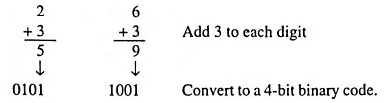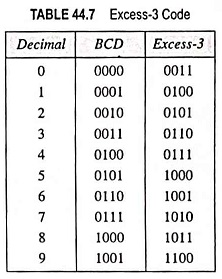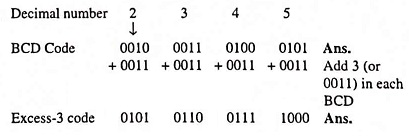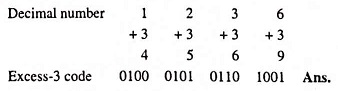## What is Excess-3 Code? (Definition and Examples)

The excess-3 code, abbreviated as XS-3, is an important 4-bit code sometimes used with binary-coded decimal (BCD) numbers. It possesses advantages in certain arithmetic operations.

The excess-3 code for a decimal number can be obtained in the same manner as BCD except that 3 is added to each decimal digit before encoding it in binary. For example, to encode the decimal digit 5 into excess-3 code, we must first add 3 to obtain 8. The digit 8 is encoded in its equivalent 4-bit binary code 1000. As another example, let us convert 26 into its excess-3 code representation.Since no definite weights can be assigned to the four digit positions, excess-3 is an unweighted code. Excess-3 codes for decimal digits 0 through 9 are given in Table 44.7. The noteworthy point from the Table 44.6 is that both codes (BCD and excess-3) use only 10 of the 16 possible 4-bit code groups. The excess-3 codes, however, does not use the same code groups. For excess-3 codes, the invalid code groups are 0000, 0001, 0010, 1101, 1110 and 1111.The key feature of the excess-3 codes is that it is self-complementing code. It means that 1’s complement of the coded number yields 9’s complement of the number itself. For example, excess-3 code of decimal 5 is 1000, its 1’s complement is 0111, which is excess-3 code for decimal 4, which is 9’s complement of 5.

It should be noted that the 1’s complement is easily produced with digital logic circuits by simply inverting each bit. The self-complementing property makes the excess-3 code useful in some arithmetic operations, because subtraction can be performed using the 9’s complement method.

Example 44.70: Encode the decimal number 2345 in BCD and excess-3 codes.

Solution:Example 44.71: Encode (1236)10 In excess-3 code.

Solution:Scroll to Top